# JP4905457B2 - Radar target detection method and radar apparatus using the target detection method - Google Patents

## Info

Publication number
JP4905457B2
JP4905457B2 JP2008542029A JP2008542029A JP4905457B2 JP 4905457 B2 JP4905457 B2 JP 4905457B2 JP 2008542029 A JP2008542029 A JP 2008542029A JP 2008542029 A JP2008542029 A JP 2008542029A JP 4905457 B2 JP4905457 B2 JP 4905457B2
Authority
JP
Japan
Prior art keywords
antenna elements
direction
target
plurality
θ
Prior art date
Legal status (The legal status is an assumption and is not a legal conclusion. Google has not performed a legal analysis and makes no representation as to the accuracy of the status listed.)
Expired - Fee Related
Application number
JP2008542029A
Other languages
Japanese (ja)
Other versions
JPWO2008053685A1 (en
Inventor

Original Assignee

Priority date (The priority date is an assumption and is not a legal conclusion. Google has not performed a legal analysis and makes no representation as to the accuracy of the date listed.)
Filing date
Publication date
Priority to JP2006297475 priority Critical
Priority to JP2006297475 priority
Application filed by 株式会社村田製作所 filed Critical 株式会社村田製作所
Priority to PCT/JP2007/069954 priority patent/WO2008053685A1/en
Priority to JP2008542029A priority patent/JP4905457B2/en
Publication of JPWO2008053685A1 publication Critical patent/JPWO2008053685A1/en
Application granted granted Critical
Publication of JP4905457B2 publication Critical patent/JP4905457B2/en
Expired - Fee Related legal-status Critical Current
Anticipated expiration legal-status Critical

• 238000001514 detection method Methods 0.000 title claims description 30
• 230000000051 modifying Effects 0.000 claims description 58
• 238000004364 calculation methods Methods 0.000 description 18
• 238000000034 methods Methods 0.000 description 4
• 230000000694 effects Effects 0.000 description 2
• 238000005070 sampling Methods 0.000 description 1

## Images

•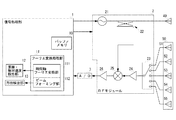•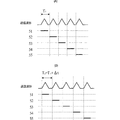•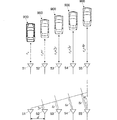•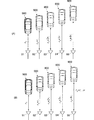•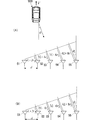•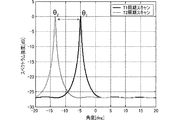•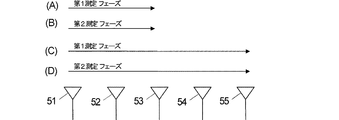•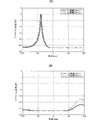•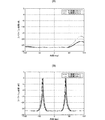•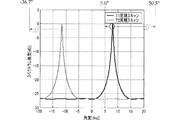•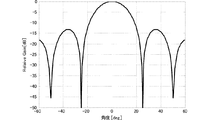•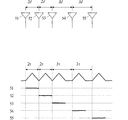•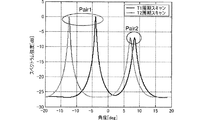•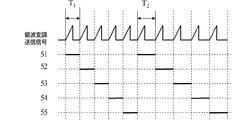•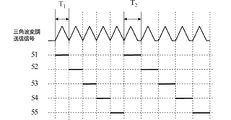••## Classifications

• GPHYSICS
• G01MEASURING; TESTING
• G01S13/00Systems using the reflection or reradiation of radio waves, e.g. radar systems; Analogous systems using reflection or reradiation of waves whose nature or wavelength is irrelevant or unspecified
• G01S13/02Systems using reflection of radio waves, e.g. primary radar systems; Analogous systems
• G01S13/06Systems determining position data of a target
• G01S13/42Simultaneous measurement of distance and other co-ordinates
• GPHYSICS
• G01MEASURING; TESTING
• G01S13/00Systems using the reflection or reradiation of radio waves, e.g. radar systems; Analogous systems using reflection or reradiation of waves whose nature or wavelength is irrelevant or unspecified
• G01S13/02Systems using reflection of radio waves, e.g. primary radar systems; Analogous systems
• G01S13/06Systems determining position data of a target
• G01S13/08Systems for measuring distance only
• G01S13/32Systems for measuring distance only using transmission of continuous unmodulated waves, amplitude-, frequency- or phase-modulated waves
• G01S13/34Systems for measuring distance only using transmission of continuous unmodulated waves, amplitude-, frequency- or phase-modulated waves using transmission of frequency-modulated waves and the received signal, or a signal derived therefrom, being heterodyned with a locally-generated signal related to the contemporaneous transmitted signal to give a beat-frequency signal
• G01S13/343Systems for measuring distance only using transmission of continuous unmodulated waves, amplitude-, frequency- or phase-modulated waves using transmission of frequency-modulated waves and the received signal, or a signal derived therefrom, being heterodyned with a locally-generated signal related to the contemporaneous transmitted signal to give a beat-frequency signal using sawtooth modulation
• GPHYSICS
• G01MEASURING; TESTING
• G01S13/00Systems using the reflection or reradiation of radio waves, e.g. radar systems; Analogous systems using reflection or reradiation of waves whose nature or wavelength is irrelevant or unspecified
• G01S13/02Systems using reflection of radio waves, e.g. primary radar systems; Analogous systems
• G01S13/06Systems determining position data of a target
• G01S13/08Systems for measuring distance only
• G01S13/32Systems for measuring distance only using transmission of continuous unmodulated waves, amplitude-, frequency- or phase-modulated waves
• G01S13/34Systems for measuring distance only using transmission of continuous unmodulated waves, amplitude-, frequency- or phase-modulated waves using transmission of frequency-modulated waves and the received signal, or a signal derived therefrom, being heterodyned with a locally-generated signal related to the contemporaneous transmitted signal to give a beat-frequency signal
• G01S13/345Systems for measuring distance only using transmission of continuous unmodulated waves, amplitude-, frequency- or phase-modulated waves using transmission of frequency-modulated waves and the received signal, or a signal derived therefrom, being heterodyned with a locally-generated signal related to the contemporaneous transmitted signal to give a beat-frequency signal using triangular modulation
• GPHYSICS
• G01MEASURING; TESTING
• G01S13/00Systems using the reflection or reradiation of radio waves, e.g. radar systems; Analogous systems using reflection or reradiation of waves whose nature or wavelength is irrelevant or unspecified
• G01S13/02Systems using reflection of radio waves, e.g. primary radar systems; Analogous systems
• G01S13/06Systems determining position data of a target
• G01S13/46Indirect determination of position data
• GPHYSICS
• G01MEASURING; TESTING
• G01S13/00Systems using the reflection or reradiation of radio waves, e.g. radar systems; Analogous systems using reflection or reradiation of waves whose nature or wavelength is irrelevant or unspecified
• G01S13/02Systems using reflection of radio waves, e.g. primary radar systems; Analogous systems
• G01S13/50Systems of measurement based on relative movement of target
• G01S13/58Velocity or trajectory determination systems; Sense-of-movement determination systems
• G01S13/583Velocity or trajectory determination systems; Sense-of-movement determination systems using transmission of continuous unmodulated waves, amplitude-, frequency-, or phase-modulated waves and based upon the Doppler effect resulting from movement of targets
• GPHYSICS
• G01MEASURING; TESTING
• G01S13/00Systems using the reflection or reradiation of radio waves, e.g. radar systems; Analogous systems using reflection or reradiation of waves whose nature or wavelength is irrelevant or unspecified
• G01S13/93Radar or analogous systems specially adapted for specific applications for anti-collision purposes
• G01S13/931Radar or analogous systems specially adapted for specific applications for anti-collision purposes of land vehicles
• GPHYSICS
• G01MEASURING; TESTING
• G01S13/00Systems using the reflection or reradiation of radio waves, e.g. radar systems; Analogous systems using reflection or reradiation of waves whose nature or wavelength is irrelevant or unspecified
• G01S13/93Radar or analogous systems specially adapted for specific applications for anti-collision purposes
• G01S13/931Radar or analogous systems specially adapted for specific applications for anti-collision purposes of land vehicles
• G01S2013/9327Sensor installation details
• G01S2013/93271Sensor installation details in the front of the vehicles

## Description

The present invention relates to a radar target detection method, particularly to a target relative speed detection method, and a radar apparatus using the detection method.

Conventionally, various radar devices have been devised that are provided on the front side of an automobile, transmit a transmission wave to a predetermined detection area including the front of the automobile, receive a reflected wave of a target in the detection area, and detect the target. Has been. As such a radar apparatus, the FMCW system is often used in the automobile field.

For example, as shown in Patent Literature 1 and Patent Literature 2, an FMCW radar device alternately alternates an upstream modulation section in which the frequency of the transmission signal gradually increases and a downstream modulation section in which the frequency of the transmission signal gradually decreases. The provided triangular wave-shaped transmission signal is used. The radar apparatus calculates the beat frequency in the upstream modulation section and the beat frequency in the downstream modulation section. Here, the beat frequency is a frequency obtained by mixing the frequency of the transmission signal and the frequency of the reception signal with respect to the transmission signal. The radar apparatus calculates the relative velocity of the target from the difference value between the beat frequency in the upstream modulation section and the beat frequency in the downstream modulation section.

Japanese Patent No. 3622565 Japanese Patent No. 3575694

However, in the methods of Patent Documents 1 and 2, when calculating the relative speed, the beat frequency and the Doppler frequency must be calculated, which complicates the processing.
Also, if there are multiple targets in the detection area, there will be multiple beat frequency spectrum peaks and it is necessary to pair the corresponding spectra. May occur. If a pairing error occurs, the relative speed cannot be calculated accurately.

Accordingly, an object of the present invention is to provide a radar target detection method capable of detecting the relative speed of a target with high accuracy without performing complicated calculation processing such as Doppler frequency for the target target, and this method. It is an object of the present invention to provide a radar device that detects a target by using it.

Another object of the present invention is to provide a radar target detection method capable of detecting the direction of a target substantially simultaneously with the detection of the relative velocity as described above, and a radar apparatus for detecting a target using this method. Is to provide.

(A) In the present invention, at least one of the transmission antenna and the reception antenna is constituted by a plurality of antenna elements arranged on a straight line, and the plurality of antenna elements arranged on the straight line are synchronized with the modulation period of the transmission signal. The present invention relates to a radar target detection method to be switched and a radar apparatus using the target detection method. The target detection method includes a first measurement phase in which a plurality of antenna elements are switched at a first time interval based on a predetermined switching pattern set in advance to calculate a first orientation of the target, and a plurality of antenna elements Is switched at a second time interval different from the first time interval based on a predetermined switching pattern, and has a second measurement phase for calculating the second orientation of the target. Further, the target detection method calculates the relative velocity of the target based on the first azimuth, the second azimuth, the first time interval, the second time interval, and the arrangement interval of the plurality of antenna elements. It is a feature.

In the case of such a radar, either one or both of a transmission antenna and a reception antenna are composed of a plurality of antenna elements arranged on a straight line, and target detection is performed while switching antenna elements for transmission and reception. Specifically, when a transmission / reception system is configured with a transmission antenna composed of one antenna element and a reception antenna in which a plurality of antenna elements are arranged on a straight line, the reflected wave based on the transmission wave from the transmission antenna is switched and controlled. The received signals are sequentially received by the antenna elements of the received antennas. In addition, when a transmission / reception system is configured with a transmission antenna in which a plurality of antenna elements are arranged on a straight line and a reception antenna composed of one antenna element, transmission waves are sequentially transmitted from each antenna element of the transmission antenna controlled to be switched. Then, a reflected wave based on each transmission wave is received by the reception antenna to generate a reception signal. In addition, when a transmission / reception system is configured by configuring both a transmission antenna and a reception antenna from a plurality of antenna elements arranged on a straight line, transmission waves are sequentially transmitted from each antenna element of the transmission antenna controlled to be switched. Then, the reception signal is generated for each combination of the antenna element of the transmission antenna and the antenna element of the reception antenna by sequentially receiving the reflected wave by each antenna element of the reception antenna subjected to another switching control.

When the reception signal is obtained by performing such switching control, and the first measurement phase and the second measurement phase at which the reception signal can be obtained at different time intervals, the target has a relative velocity with respect to the radar. If so, the phase change amount of the received signal can be obtained in each phase. Due to the difference in the amount of phase change, the azimuth obtained by the beam forming method or the like differs in each phase. Here, a specific relationship is established between the difference in time interval between phases (interval time difference), the difference in azimuth calculated in each phase, the antenna element, and the relative velocity. Is calculated. Thus, the relative speed can be calculated without calculating the Doppler frequency. At this time, the true direction of the target can be calculated at the same time by using the direction calculated in each phase.

(B) According to the radar target detection method of the present invention, the direction perpendicular to the arrangement direction of the plurality of antenna elements on the radiation direction side of the transmission signal is the 0 ° direction, and the angle extends from the 0 ° direction to the arrangement direction. In the range of −90 ° to + 90 °, the first direction and the second direction are respectively θ 1 and θ 2 , the time difference between the first time interval and the second time interval is Δt, and a plurality of antennas the spacing of the elements d, as V relative speed candidate of the target, the n is an arbitrary integer, the wavelength of the transmission signal as a lambda,
Formula V = d · (sin θ 1 −sin θ 2 ) / (2 · Δt) + n · λ / (2 · Δt) − (1)
The relative speed of the target is calculated by selecting one of the candidates obtained by using.

In this method and the configuration that implements this method, the relative velocity V is calculated by using a simple equation as specifically shown in equation (1).

(C) In the radar target detection method of the present invention, the range of the relative speed that can be taken by the target target is set to V min to V max , and the interval time difference between the first time interval and the second time interval is determined. Let Δt be the wavelength of the transmitted / received signal λ,
Δt <λ / (2 | V max -V min |) - (2)
The difference Δt between the first time interval and the second time interval is set so that

In this method and the configuration that realizes this method, the minimum value and the maximum value of the relative speed that can be taken by the target are set in advance to V min to V max , and antenna element switching is performed using equation (2). An interval time difference, that is, an interval time difference Δt between the time interval of the first measurement phase and the time interval of the second measurement phase is determined. By determining the interval time difference Δt in this way, among the candidates for the relative speed obtained by the equation (1), the one in the range of V min to V max is unique. Measured reliably.

(D) In the radar target detection method of the present invention, the direction perpendicular to the arrangement direction of the plurality of antenna elements on the radiation direction side of the transmission signal is set to 0 °, and the angle range extends from the 0 ° direction to the arrangement direction. Then, the detection azimuth angle range θ min to θ max is set within the range of −90 ° to + 90 °, the arrangement interval of the plurality of antenna elements is d, and the wavelength of the transmission / reception signal is λ,
d <λ / | sin θ max −sin θ min | − (3)
The arrangement interval of a plurality of antenna elements is set so that

In this method and the configuration that realizes this method, the azimuth angle range in which target detection is performed is set in advance to θ min to θ max, and the arrangement interval d of the plurality of antenna elements is determined using Equation (3). Is done. By determining the arrangement interval d of the antenna elements in this way, the relative speed of the target within the target detection azimuth range to be measured is reliably measured, and the azimuth is uniquely detected.

(E) In the radar target detection method of the present invention, the direction perpendicular to the arrangement direction of the plurality of antenna elements on the radiation direction side of the transmission signal is defined as 0 ° direction, and the calculated azimuth angle range including the 0 ° direction is determined. −θ cal to + θ cal and the arrangement interval of the plurality of antenna elements is set to d and the wavelength of the transmission / reception signal is set to λ so that the relationship θ cal = sin −1 (λ / (2d)) is established. Thus, the relative speed and direction of the target are calculated.

In this method and the configuration that realizes this method, only one spectrum peak in each of the first measurement phase and the second measurement phase is detected by limiting the calculated azimuth angle range −θcal to + θcal by the equation (4). . Thereby, the first azimuth and the second azimuth used for calculating the relative speed are uniquely determined, and the relative speed is reliably calculated.

(F) According to the radar target detection method of the present invention, when the arrangement interval d of the plurality of antenna elements is set to be less than 0.5λ with respect to the wavelength λ of the transmission / reception signal, the antenna elements are arranged when calculating each direction A temporary azimuth is calculated by virtually setting the interval d to 0.5λ or more, and the azimuth is calculated by correcting the calculated azimuth to a setting state of less than 0.5λ.

When the antenna element interval d is less than 0.5λ, the range in which the phase difference between the antenna elements caused by the true orientation of the detection target can be taken is narrower than −λ / 2 to + λ / 2. Depending on the speed, there may be a problem that even if the arrival angle calculation is performed, the observation peak that should appear in any direction does not appear. That is, in each of the above-described arithmetic expressions, an accurate azimuth cannot be calculated practically unless the antenna element interval d is 0.5λ or more.

Therefore, in this method and the configuration that realizes this method, when calculating the detection direction, the first direction and the second direction are assumed by the above-described method, assuming that the antenna element disposition interval d is virtually 0.5λ or more. Is calculated. Then, the calculated first azimuth and second azimuth are corrected based on the relationship between the setting value of the antenna element arrangement interval d at the time of calculating the detection azimuth and the actual antenna element arrangement interval d. Thereby, the actual first azimuth and second azimuth are calculated, and the relative speed is also calculated accordingly.

(G) The radar target detection method of the present invention is characterized in that a plurality of antenna elements are arranged at unequal intervals, and the greatest common value of the unequal intervals is made to coincide with the arrangement interval d.

In this method and the configuration that realizes this method, by arranging the antenna elements at unequal intervals, the distance between both ends of the arranged antenna elements is widened, and the azimuth resolution is improved, so that the spectrum peak becomes sharper and higher. The first azimuth and the second azimuth are calculated with accuracy, and as a result, the relative speed is calculated with higher accuracy.

(H) The radar target detection method of the present invention is characterized in that the interval time difference Δt between the first time interval and the second time interval is variably set.

In this method and the configuration that implements this method, even if there are a plurality of spectrum peaks, the first direction and the second direction are associated with each other according to the spectrum intensity, so that a plurality of detection directions can be calculated simultaneously. Can do. Further, when the target for detecting the relative speed is determined, the speed resolution can be improved.

According to the present invention, the relative speed can be easily calculated by simple calculation processing without performing complicated calculation processing such as Doppler frequency. At this time, the azimuth can be calculated almost simultaneously in the same processing system.

Further, according to the present invention, the relative velocity of the target can be calculated with higher accuracy and reliability by appropriately setting the antenna interval and the detection azimuth range.

Further, according to the present invention, the relative speed calculation load can be reduced by appropriately setting the calculated azimuth angle range.

Further, according to the present invention, the relative speed detection of the target can be performed with higher accuracy by setting the antenna intervals to unequal intervals.

Moreover, according to this invention, the relative speed detection of a some target can be performed simultaneously.

It is a block diagram which shows schematic structure of the FMCW system radar apparatus of 1st Embodiment. It is explanatory drawing which shows the synchronous state of transmission control and switching control in 1st Embodiment. The change in the distance to the target due to the relative speed is shown. It is a figure which shows the change of the distance to the target by the relative speed in a 1st measurement phase, and the figure which shows the change of the distance to the target by the relative speed in a 2nd measurement phase. It is a figure which shows the change of the distance to the target in a 1st measurement phase, and the figure which shows the change of the distance to the target in a 2nd measurement phase. It is the figure which showed the azimuth direction spectrum by the received signal in the above-mentioned 1st measurement phase, and the azimuth direction spectrum by the received signal in the 2nd measurement phase. It is a figure showing the switching pattern according to the switching mode of an antenna element. It is a figure which shows an azimuth direction spectrum. It is a figure which shows an azimuth direction spectrum. It is a figure which shows an example of the azimuth | direction direction spectrum obtained by the structure and process of 2nd Embodiment. It is the figure which showed the transmission / reception beam pattern of 2nd Embodiment. It is a figure which shows an antenna element space | interval, and explanatory drawing which shows the synchronous state of transmission control and switching control. It is a figure which shows an azimuth | direction direction spectrum in case a some target exists in a different azimuth | direction at the same distance and has a different relative velocity. It is explanatory drawing which shows the synchronizing state of another transmission signal waveform and transmission control, and switching control. It is explanatory drawing which shows the synchronizing state of another transmission signal waveform and transmission control, and switching control. It is explanatory drawing which shows the synchronizing state of another transmission signal waveform and transmission control, and switching control. It is explanatory drawing which shows the synchronizing state of another transmission signal waveform and transmission control, and switching control.

A radar apparatus and a target detection method for a radar apparatus according to a first embodiment of the present invention will be described with reference to the drawings. In the present embodiment, an FMCW radar device will be described as an example of a radar device including a transmission antenna including one antenna element and a reception antenna including a plurality of antenna elements. Further, in the following description, an FM-CW radar device using a transmission signal subjected to triangular wave modulation including an upstream modulation section in which the frequency gradually increases and a downstream modulation section in which the frequency gradually decreases is shown as an example. However, the following configuration and processing can also be applied to a radar apparatus that includes only uplink modulation or only downlink modulation.

FIG. 1 is a block diagram showing a schematic configuration of the FMCW radar device of the present embodiment.
As shown in FIG. 1, the radar apparatus according to the present embodiment includes a signal processing unit 1 that performs transmission signal control, switching control, and object detection, an RF module 2, a transmission antenna 40, and a reception antenna 50.

The signal processing unit 1 generates a transmission control signal and a switching control signal as transmission system control. The transmission control signal is a signal given to the VCO 21 of the RF module 2 in order to generate a transmission signal whose frequency is modulated in a triangular shape in time series (hereinafter simply referred to as “triangular wave modulation transmission signal”). The switching control signal is a signal given to the output switching circuit 23 of the RF module 2 in order to select the antenna elements 51 to 55 of the receiving antenna 50.

The signal processing unit 1 outputs a transmission control signal so that a transmission signal is generated at different transmission periods in the first measurement phase and the second measurement phase. At this time, the generated triangular wave modulation transmission signal includes a triangular wave modulated triangular wave modulation section and a non-modulation section between the triangular wave modulation sections. The time length of the triangular wave modulation section is the same in the first measurement phase and the second measurement phase, but the non-modulation section is set so that the second measurement phase is longer than the first measurement phase. .

Figure 2 is an explanatory diagram showing a synchronization state between the transmission control and the switching control, (A) in the case of the first measurement phase is switched by the switching time T 1, (B) the second of switching in the switching time T 2 The case of the measurement phase is shown. The second measurement phase switching time T 2 is a time obtained by adding a predetermined time Δt to the first measurement phase switching time T 1 . That is, the relationship is T 2 = T 1 + Δt.

Simultaneously with such transmission control processing, the signal processing unit 1 causes the rising timing of each triangular wave modulation section and the switching timing of the antenna elements 51 to 55 so that each antenna element 51 to 55 sequentially corresponds to each triangular wave modulation section. A switching control signal for matching is output.

At this time, the switching pattern of the antenna elements 51 to 55 is a preset pattern that is the same in the first measurement phase and the second measurement phase. For example, as shown in FIG. 2, a switching pattern that repeats antenna element 51 → antenna element 52 → antenna element 53 → antenna element 54 → antenna element 55 in time series order is used. The transmission pattern of the transmission signal and the switching pattern of the antenna element are not limited to this, and various patterns described at the end of the embodiment of the present specification may be repeated.

The RF module 2 includes a VCO 21 and a distributor 22 as a transmission system circuit, and includes an output switch 23, an RF amplifier 24, a mixer 25, and an IF amplifier 26 as a reception system circuit.
As a transmission system of the RF module 2, the VCO 21 includes a so-called voltage-controlled oscillator, receives a transmission control signal from the signal processing unit 1, generates a triangular wave modulated transmission signal, and outputs it to the distributor 22.

The distributor 22 is formed of a directional coupler, and supplies the triangular wave modulated transmission signal from the VCO 21 to the transmission antenna 40, generates a local signal obtained by power distribution of the triangular wave modulated transmission signal, and supplies the local signal to the mixer 25.

The transmission antenna 40 is composed of a single patch antenna or the like, and radiates a transmission wave obtained by converting a triangular wave modulated transmission signal into a radio wave in the detection region.

The receiving antenna 50 includes antenna elements 51 to 55. The antenna elements 51 to 55 are composed of patch antennas or the like, and are composed of, for example, a plurality of patch electrodes arranged on a dielectric substrate in a straight line at equal intervals. The arrangement direction of the antenna elements 51 to 55 is a direction perpendicular to the front direction of the radar apparatus (the front direction of the automobile in which the radar apparatus is installed) and along the horizontal direction. In this embodiment, as a more specific positional relationship, it is assumed that antenna elements 51, 52, 53, 54, and 55 are arranged in order from the right end to the left end when viewed from the front of the radar apparatus.

The antenna elements 51 to 55 of the reception antenna 50 receive a reflected wave of a target based on the transmission wave, generate a reception signal, and output the reception signal to the output switch 23 of the RF module 2.

As a reception system of the RF module 2, the output switch 23 receives reception signals from the antenna elements 51 to 55. The output switching device 23 is supplied with the switching control signal described above, and the output switching device 23 switches the connection between any of the antenna elements 51 to 55 and the RF amplifier 24 based on the switching control signal. That is, the reception signal of the antenna element selected by the switching control signal is given to the RF amplifier 24. At this time, switching of the antenna element is performed in accordance with the transmission cycle T 1 in the first measurement phase, and is performed in accordance with the transmission cycle T 2 in the second measurement phase, and the output is selected for each of the aforementioned triangular wave modulation sections. A reception signal of the antenna element is given to the RF amplifier 24.

The RF amplifier 24 performs gain control of the given received signal and outputs the RF signal after gain control to the mixer 25.
The mixer 25 multiplies the RF signal and the local signal to generate an IF beat signal and supplies the IF beat signal to the IF amplifier 26. The IF amplifier 26 performs gain control of the IF beat signal and outputs it to the A / D converter 3.

The A / D converter 3 samples the IF beat signal after amplification (gain control) at a predetermined sampling period, thereby converting the analog IF beat signal into a digital IF beat signal and outputting the digital IF beat signal to the signal processing unit 1. .

The buffer memory 10 which is a reception system of the signal processing unit 1 sequentially buffers the input IF beat signal. At this time, the IF beat signal is buffered in the buffer memory 10 in units of measurement phases.

The Fourier transform processing unit 11 includes a time axis Fourier transform unit 111 and a beam forming unit 112. The time-axis Fourier transform unit 111 generates a frequency spectrum using a known FFT process and supplies the frequency spectrum to the distance / relative velocity detection unit 12. The beam forming unit 112 generates a azimuth direction spectrum by applying a known beamformer method or Capon method using the frequency spectrum generated by the time-axis Fourier transform unit, and supplies the azimuth direction spectrum to the azimuth detection unit 13. give. At this time, the Fourier transform processing unit 11 generates an azimuth direction spectrum in units of measurement phases.

The distance / relative speed detection unit 12 calculates the relative speed of the detected target with respect to the own apparatus using the following method.

[Description of the principle of relative speed detection]
The radar apparatus according to the present embodiment detects the relative speed of the target based on the following principle.
3 to 7 are diagrams for explaining the principle of detecting the target relative speed, and FIG. 3 shows a change in the distance to the target due to the relative speed. In FIG. 3, d represents the arrangement interval of the antenna elements, r 0 represents the distance between the target 900 and the antenna element 51 when the received signal of the antenna element 51 is output selected, and r represents the antenna switching period. It represents the distance change due to relative speed.

As shown in FIG. 3, when the target 900 is performing a constant velocity linear motion that is not “0” with respect to the own device, in one measurement phase, when the distance when the antenna element 51 is selected is r 0 , The distance when the antenna element 52 is selected is r 0 + r, the distance when the antenna element 53 is selected is r 0 + 2r, the distance when the antenna element 54 is selected is r 0 + 3r, and the distance when the antenna element 55 is selected is r 0. + 4r. Therefore, assuming that the front direction of the receiving antenna 50 is 0, the azimuth angle based on the relative velocity is θ V , and the antenna element spacing is d, an equiphase surface is generated in the direction of θ V = sin −1 (2r / d). Is equivalent to This corresponds to the deviation of the azimuth angle due to the relative speed in the positioning phase.

In such a situation, if the transmission period (switching period) is different between the first measurement phase (transmission period T 1 ) and the second measurement phase (transmission period T 2 ), the distance when each antenna element is selected is as shown in FIG. The phase relationship as shown in FIG.

FIG. 4A is a diagram illustrating a change in the distance to the target due to the relative speed in the first measurement phase, and FIG. 4B is a distance to the target due to the relative speed in the second measurement phase. It is a figure which shows the change of.

As shown in FIG. 4A, in the first measurement phase, the distance changes every r 1 according to the transmission cycle T 1 . Specifically, when the distance at the time of selection of the antenna element 51 and r 0, the distance at the time of selection of the antenna element 52 is r 0 + r 1, the distance at the time of selection of the antenna element 53 r 0 + 2r 1, antenna element 54 The distance when selecting is r 0 + 3r 1 , and the distance when selecting the antenna element 55 is r 0 + 4r 1 .

On the other hand, as shown in FIG. 4B, in the second measurement phase, the distance changes every r 2 according to the transmission cycle T 2 . Specifically, when the distance at the time of selection of the antenna element 51 and r 0, the antenna element distance when selecting the 52 r 0 + r 2, the distance at the time of selection of the antenna element 53 r 0 + 2r 2, antenna element 54 The distance when selecting is r 0 + 3r 2 , and the distance when selecting the antenna element 55 is r 0 + 4r 2 . Here, r 2 = r 1 + Δr, and Δr is a distance difference according to the time length difference Δt between the transmission periods T 1 and T 2 .

When a change in distance due to the speed of the target is observed between the antenna elements in this way, the change in the distance between the radar apparatus and the target as well as the distance change due to the direction of the target with respect to the radar apparatus is as shown in FIG. Become.
FIG. 5A is a diagram illustrating a change in the round-trip distance from the radar apparatus to the target in the first measurement phase, and FIG. 5B is a radar apparatus to the target in the second measurement phase. It is a figure which shows the change of the round-trip distance from.

In the first measurement phase, as specifically shown in FIG. 5A, when the distance from the antenna element 51 to the virtual phase reference plane is “0”, each antenna element 52, 53, 54, 55 The distances to the virtual phase reference plane are L + 2r 1 , 2L + 4r 1 , 3L + 6r 1 , 4L + 8r 1 , respectively. Here, L is the distance change due to the azimuth θ of the target, and 2r 1 is the distance change due to the velocity V of the target.

Further, in the second measurement phase following the first measurement phase, as shown in FIG. 5B, when the distance from the antenna element 51 to the virtual phase reference plane is “0”, each antenna element The distances from 52, 53, 54, 55 to the virtual phase reference plane are L + 2r 2 , 2L + 4r 2 , 3L + 6r 2 , 4L + 8r 2 , respectively. Here, L is the distance change due to the azimuth θ of the target, and 2r 2 is the distance change due to the velocity V of the target.

From this relationship, the first direction calculated in the first measurement phase is θ 1 , the second direction calculated in the second measurement phase is θ 2, and the true direction that is not affected by the relative speed is θ If the deviation of the first measurement phase orientation relative to the true orientation θ is θ r1 and the orientation deviation of the second measurement phase orientation relative to the true orientation θ is θ r2 , then the following two equations are obtained.

L + 2r 1 = −d (sin θ + sin θ r1 ) = − d · sin θ 1 − (5)
L + 2r 2 = −d (sin θ + sin θ r2 ) = − d · sin θ 2 − (6)
As for the sign of the azimuth, the directions of θ 1 and θ 2 shown in FIG. 5 are defined as negative.

Here, if defined as Δr = r 2 −r 1 ,

It becomes. In other words, since the equation (7) is the first term on the right side of the equation (1) representing the relative velocity candidate V, the relative velocity candidate V of the target can be calculated by performing this calculation process. it can.

The signal processing unit 1 calculates the relative speed candidate V according to the following processing flow.

First, the signal processing unit 1 calculates the azimuth directional spectrum from the received signal group of the first measuring phase, and calculates the sine value sin [theta 1 of the azimuth angle theta 1. Then, the signal processing unit 1 calculates the azimuth directional spectrum from the received signal group in the second measurement phase following the first measuring phase, and calculates the sine value sin [theta 2 of the azimuth angle theta 2. Then, the signal processing unit 1 calculates the relative speed candidate V by applying the calculated sin θ 1 and sin θ 2 to the equation (1).

As a specific example, the number of receiving antenna elements is 5, the antenna interval is 1.46λ (λ is the wavelength of a 76 GHz transmission signal), and the antenna switching period T 1 in the first measurement phase is 1 msec. And the antenna switching period T 2 in the second measurement phase is 1.05 msec. FIG. 6 shows the azimuth direction spectrum when the simulation is performed using the Capon method when the target orientation is 5 ° and the relative velocity of the target is 30 km / h. FIG. 6 is a diagram showing the azimuth direction spectrum by the received signal in the first measurement phase and the azimuth direction spectrum by the received signal in the second measurement phase. In FIG. 6 and subsequent figures, the case where the T 1 period scan in the drawing is the first measurement phase is shown, and the case where the T 2 period scan is the second measurement phase is shown.

As shown in FIG. 6, the orientation theta 1 of the first measuring phase for switching the order of the antenna elements 51 to 55 at the switching period T 1 switches sequentially with -5.00 °, and the switching period T 2 The direction θ 2 in the second measurement phase is −13.47 °. Therefore, the calculated relative speed candidate is 30.0 ± 141.1 × n km / h from the equation (1), and the value when n = 0 matches the simulation assumption. If the speed that the target can take is -40 km / h to 100 km / h, the true relative speed can be specified to be 30 km / h.

As described above, by using the configuration and processing of the present embodiment, the radar apparatus of the present embodiment reliably ensures the relative speed of the target without performing complicated processing such as calculation of the Doppler frequency of the target. It can be detected well. Moreover, since the processing calculation is simple, highly accurate detection can be performed at high speed.

In the above description, the first measurement phase and the second measurement phase are configured using all the antenna elements 51 to 55 configuring the receiving antenna. However, all the antenna elements from one end to the other end of the antenna array are not necessarily configured. It is not necessary to switch to the antenna element, and a pattern for switching a part of the antenna elements as shown in FIG. 7 may be used. FIG. 7 is a diagram showing switching patterns for each antenna element switching mode. (A) and (B) show the high-speed detection mode, and (C) and (D) show the normal detection mode.

The signal processing unit 1 is provided with a mode switching function. For example, the mode is switched by an operation input from the user. Here, the modes include, for example, a high-speed detection mode that detects a relative speed at a high speed and a normal detection mode that detects a relative speed in a normal detection time. When receiving the selection of the high-speed detection mode, the signal processing unit 1 detects the relative speed from the first measurement phase shown in FIG. 7A and the second measurement phase shown in FIG. 7B. That is, first, as the first measurement phase, the first azimuth θ 1 is calculated by switching the three antenna elements 51 to 53 at the switching period T 1 . Thereafter, a second measuring phase, calculates a second orientation theta 2 switches the three antenna elements in the switching period T 2 of the antenna elements 51-53. Then, the relative velocity V is calculated using the first azimuth θ 1 and the second azimuth θ 2 . In this method, since the number of switching antenna elements is small, the relative speed can be detected at a higher speed than in the normal detection mode.

On the other hand, when receiving the selection of the normal detection mode, the signal processing unit 1 detects the relative speed from the first measurement phase shown in FIG. 7C and the second measurement phase shown in FIG. That is, first, as a first measurement phase, calculates a first orientation theta 1 all antenna elements are switched by the switching period T 1 of the antenna elements 51-55. Thereafter, a second measuring phase, calculates a second orientation theta 2 is switched every antenna element in the switching period T 2 of the antenna elements 51-55. Then, the relative velocity V is calculated using the first azimuth θ 1 and the second azimuth θ 2 . In this method, since the number of switching antenna elements is larger than in the high-speed detection mode, the relative speed can be detected with higher accuracy than in the high-speed detection mode.

By the way, in the above description, the time range is not specified for the antenna element switching interval difference Δt. However, by setting the switching interval difference Δt by the following method, the relative speed candidate V appearing within the range of the relative speed that can be taken by the target is unique, and the relative speed can be calculated without ambiguity. it can.
When the relative speed of the target increases, the distance difference Δr with respect to the time difference (switching interval difference) Δt between the switching period T 1 of the first measurement phase and the switching period T 2 of the second measurement phase also increases. A phase difference corresponding to the reciprocal distance difference 2Δr is generated between the first measurement phase and the second measurement phase. At this time, if the wavelength of the transmission signal is λ and the phase difference corresponding to the distance difference 2Δr exists within −λ / 2 to + λ / 2, ambiguity does not occur and the relative velocity V is calculated reliably. can do. That is, the absolute value of the phase difference due to 2Δr may be λ / 2 or less. Here, candidates for the relative velocity V appear at intervals of λ / (2Δt). Therefore, when the maximum relative speed candidate in the candidate range of the relative speed V is V max and the minimum relative speed candidate is V min , the relative speed candidate is uniquely determined as follows:

This is the case where For this reason,

And the relative speed can be calculated uniquely and more reliably by setting the switching interval difference Δt. Moreover, once the target is detected, an approximate value of the relative speed can be obtained. V prv is the relative velocity of the target obtained in the previous measurement, α is the maximum acceleration possible due to the nature of the target motion, -β is also the deceleration, and τ is the time difference between the previous measurement and the current measurement path. Then, the maximum value V max and the minimum value V min of the speed that the target can take at the time of this measurement can be expressed as V prv + ατ and V prv −βτ, respectively.
| V max −V min | = (V prv + ατ) − (V prv −βτ) = (α + β) τ− (9 ′)
It can be expressed as.

Here, a specific method for setting Δt will be described. First, in a state where the target is not detected, for example, assuming that the target is an automobile, V max = + 200 km / h, V min = −200 km / h, the maximum acceleration α = 5 m / s 2 , and the maximum The deceleration β is 10 m / s 2 and the time difference τ is 0.1 sec. From the equations (9) and (9 ′), the switching interval difference Δt = 1 msec. And it is sufficient.

By setting the switching interval difference Δt in this way, the relative speed V can be calculated more reliably.

Furthermore, when the antenna element interval d is 0.5λ or more, the relative speed and direction of the target can be reliably detected.

FIG. 8 is a diagram showing the azimuth direction spectrum when the antenna interval is d = 0.4λ and the true azimuth of the target is 5 °. FIG. 8A shows the relative velocity of the target at 2.0 km / h. A case is shown, and (B) shows a case where the relative velocity of the target is 4.0 km / h.
When the antenna element interval is d, the detection range of the distance difference generated between adjacent antenna elements is −d + nλ to + d + nλ (n is an integer). That is, if the distance difference obtained by adding the distance difference due to the positional relationship between the antenna element and the target and the distance difference caused by the relative speed does not fall within the range of −d + nλ to + d + nλ, the azimuth direction as shown in FIG. The spectrum peak of the spectrum cannot be obtained.

Here, for example, when the antenna element interval d is less than 0.5λ (d = 0.4λ), the distance difference detection range is −0.4λ + nλ to 0.4λ + nλ. The switching period T 1 of the first measurement phase is 1 msec. The switching period T 2 of the second measurement phase is 1.05 msec. When the relative speed of the target is 2 km / h, the distance difference between adjacent antenna elements is 0.32λ. In this case, since the above-mentioned conditions −0.4λ + nλ to 0.4λ + nλ are satisfied, a spectrum peak is obtained (see FIG. 8A), and the relative velocity can be detected.

However, when the relative speed of the target is 4 km / h, the distance change due to the relative speed between adjacent antenna elements is 0.60λ. In this case, since the above-mentioned conditions −0.4λ + nλ to 0.4λ + nλ are not satisfied, a spectrum peak cannot be obtained (see FIG. 8B), and the relative velocity cannot be accurately detected.

On the other hand, when the antenna element interval d is 0.5λ or more, for example, if d = 0.8λ, the detection range of the distance difference between adjacent antenna elements is (−0.8 + n) λ to (0.8 + n) λ. It becomes. In this case, since the range when n = m and the range when n = m + 1 overlap, all the distance differences can be detected. That is, the distance difference obtained by adding the distance difference due to the positional relationship between the antenna element and the target and the distance difference caused by the relative speed surely exists in the range of −d + nλ to + d + nλ. As a result, by setting the antenna element interval d to 0.5λ or more, a spectrum peak in the azimuth direction can be reliably obtained, and the relative velocity of the target can be detected.

In the above description, it has been shown that the spectral peak can be obtained reliably and easily by setting the antenna element interval d to 0.5λ or more. However, even if the antenna element interval d is less than 0.5λ, A spectrum peak can also be obtained by virtually setting the antenna element interval d to 0.5λ or more in the arithmetic of the Capon method and the beamformer method.

In the Capon method, Beamformer method, etc., the mode vector shown in the following equation is used for the arrival direction estimation calculation.

Here, a (θ) represents a mode vector with respect to the estimated azimuth θ, and d1 to dk represent antenna intervals when one antenna element position is a reference position in the number of antenna elements k + 1.
When the antenna element interval d is less than 0.5λ, the actual antenna element interval d ′ is used instead of the actual antenna element interval d for d1 to dk in (Formula A).

By this calculation process, an azimuth direction spectrum as shown in FIG. 9B is obtained.
9A and 9B are diagrams showing the azimuth direction spectrum, where FIG. 9A shows a case where an actual antenna element interval d = 0.4λ is used, and FIG. 9B shows a virtually set antenna element interval d ′ = 2d = 0.8λ. The case where is used is shown.
Note that the azimuth direction spectrum shown in FIG. 9 is obtained under the same conditions as the azimuth direction spectrum shown in FIG. That is, FIG. 9A and FIG. 8B show the same result.

As shown in FIG. 9B, when the antenna element interval d ′ is virtually set to 0.5λ or more, a steep spectral peak is obtained.

By virtually setting the antenna element interval to 0.5λ or more in this way, a spectrum peak can be obtained without being affected by the relative speed even if the actual antenna element interval d is less than 0.5λ. it can.

The azimuth θ 1 ′ in the first measurement phase and the azimuth θ 2 ′ in the second measurement phase based on the spectrum peak thus obtained are different from the actual azimuth θ. However, the actual azimuth θ and the calculated azimuth θ ′ are L as the distance to the equiphase plane,
L = d · sin θ = d ′ · sin θ ′
Since the relationship of
The actual orientation θ is
θ = sin −1 ((d ′ / d) · sin θ ′) − (formula B)
Can be obtained from

As described above, in reality, even if the antenna element interval d is less than 0.5λ, the number of processing operations increases by virtually setting the antenna element interval d ′ to 0.5λ or more in arithmetic operations. However, the azimuth θ can be obtained. As a result, the relative velocity V can be calculated using the calculated directions θ1 and θ2 of each measurement phase.

Next, a radar apparatus and a target detection method for the radar apparatus according to the second embodiment will be described with reference to the drawings.
The radar apparatus of this embodiment is different only in the antenna interval d, and the other configuration is the same as that of the radar apparatus of the first embodiment.
In the present embodiment, the true azimuth θ of the target is calculated together with the relative velocity V of the target, and a method for calculating the true azimuth θ will be specifically described below.

As the relative speed of the target increases, the distance difference r caused by the relative speed between adjacent antenna elements also increases. Usually, if the distance difference r caused by the relative speed is designed to fall within the range of -λ / 2 to + λ / 2, ambiguity will not occur. However, when the distance difference r due to the relative speed does not fall within the range of -λ / 2 to + λ / 2, it is impossible to distinguish between r and r + nλ (n is an integer).

In this case, if the first direction of the first measurement phase is θ 1 ,
L + 2r 1 + nλ = −d · sin θ 1 − (10)
It becomes. However, n is an arbitrary integer.

There are a plurality of azimuth candidates having such a combination. Here, the relative velocity of the target is V, and the true direction θ is
r 1 = V · T 1- (11-1)
L = −d · sin θ− (11-2)
Therefore, from the equations (10), (11-1), (11-2)

It becomes. Therefore, from equation (12), candidates for the sine value sin θ in the true direction θ are generated at intervals of λ / d.

As described above, since the sine value sin θ is generated at intervals of λ / d, in order to specify sin θ, the possible value of θ is set to be perpendicular to the front direction of the radar, that is, the arrangement direction of the plurality of antenna elements, and the transmission signal. When the transmission direction is set to 0 ° direction, θ min is the minimum value and θ max is the maximum value within the range of −90 ° to + 90 °,

It may be limited to. Thereby, a candidate is determined uniquely and a true direction can be specified.

Therefore,

By setting the antenna element interval d so as to be true, the true azimuth θ can be calculated reliably and easily. At this time, the relative velocity V can be obtained by performing the calculation of the above-described embodiment using sin θ 1 and sin θ 2 used when calculating the true direction θ.

FIG. 10 is a diagram showing an example of the azimuth direction spectrum obtained by the configuration and processing of the present embodiment. The azimuth direction spectrum shown in FIG. 10 has a transmission signal frequency of 76 GHz, a wavelength λ of 3.9 mm, and a first measurement phase switching period T 1 of 1 msec. And the switching period T 2 of the second measurement phase is 1.05 msec. And the simulation result when the target direction θ is 5 ° and the relative speed of the target is 70 km / h.

The antenna element interval d is determined to be 1.46λ from equation (14) by setting θ max to + 20 ° and θ min to −20 °, but here, d = 1.46λ is set as the boundary value. is doing. In order to realize θ max = 20 ° and θ min = −20 °, the radar apparatus of this embodiment forms, for example, a transmission / reception beam pattern having directivity characteristics as shown in FIG. FIG. 11 is a diagram showing a transmission / reception beam pattern of the present embodiment. Such a transmission / reception beam pattern can be set by the configuration of transmission antennas and reception antennas and transmission / reception control.

As shown in FIG. 10, among the θs obtained by substituting the first orientation θ 1 of the first measurement phase into the equation (12) by using the antenna element arrangement interval d of the present embodiment, θ max to There is only one in the θ min range. Similarly, there is only one θ obtained by using the second orientation θ 2 of the second measurement phase instead of θ 1 in the equation (12) in the range of θ max to θ min . The relative speed candidate V can be calculated from sin θ 1 and sin θ 2 by using equation (1) of the above-described embodiment. Specifically, in the example of FIG. 10, θ 1 = 7.96 ° and θ 2 = −11.62 °, so the relative speed candidate V is 70.0 ± 141.1 × n km / h, and n The value when = 0 matches the assumption. Now, if the speed that the target can take is -40 km / h to +100 km / h, the true relative speed can be specified to be 70 km / h.

Next, since the sine value sin θ of the true azimuth θ appears every λ / d as shown in the equation (12), from θ 1 = 7.96 °, the true azimuth θ candidate is realistically Within the range of −90 ° to + 90 ° that can be detected by the transmission signal, −36.7 °, + 5.0 °, and + 50.5 °. However, since the transmission beam range is set to −20 ° to + 20 °, the true azimuth θ is uniquely determined to be 5.0 °. This result is consistent with the assumption.

As described above, by performing the configuration and processing of the present embodiment, it is possible to reliably and accurately detect the relative speed and direction of the target.

In the present embodiment, the detection range is set to θ min to θ max . However, when both the positive direction and the negative direction of the angle are set within the same angle range with the 0 ° direction as the center, the equation (14) Instead of

May be used.

Next, a radar apparatus and a target detection method for the radar apparatus according to the third embodiment will be described with reference to the drawings.
The radar apparatus according to the present embodiment has unequal antenna element intervals, and the other configurations are the same as those of the first and second embodiments.
FIG. 12A is a diagram illustrating antenna element intervals, and FIG. 12B is an explanatory diagram illustrating a synchronization state between transmission control and switching control.

As shown in FIG. 12A, in the radar apparatus of the present embodiment, the intervals between the antenna elements 51 to 55 are set to 2d, 2d, 3d, and 3d in order from the antenna element 51 side. Then, as shown in FIG. 12B, when the signal processing unit 1 switches the antenna elements in the order of the antenna elements 51, 52, 53, 54, 55, τ = T 1, that is, 2T in the first measurement phase. Switching is performed at time intervals of 1 , 2T 1 , 3T 1 , 3T 1 , and in the second measurement phase, switching is performed at time intervals of τ = T 2, that is, 2T 2 , 2T 2 , 3T 2 , 3T 2 .

In the case of an unequally spaced array antenna, the grating lobe generation interval is determined by the greatest common divisor of the antenna element intervals. For this reason, assuming that the greatest common divisor of the antenna element spacing is d, from the conditions of the first and second embodiments described above,

If d is determined so as to satisfy, the relative velocity V and the true orientation θ can be uniquely determined. Alternatively, when d <0.5λ, θ is calculated from Equation (B) in the same manner using the above-described virtual interval d ′ (d ′> 0.5λ), and then the relative velocity V is uniquely set. Can be determined.

By using such a configuration and processing, it is possible to improve the azimuth resolution by the unequal interval array and reliably detect the relative velocity V and the true azimuth θ. That is, the relative speed V and the true orientation θ can be detected with high accuracy and reliability.

Next, a radar apparatus and a target detection method for the radar apparatus according to the fourth embodiment will be described.
In the present embodiment, an azimuth angle range (estimated calculation azimuth angle range) for estimation calculation is set, and other configurations are the same as those of the radar apparatus shown in the third embodiment.

When the estimated calculation azimuth angle range is set to -θ cal to + θ cal , the maximum calculation azimuth angle θ cal is
θ cal = sin −1 (λ / (2d)) − (17)
And

This relational expression is derived from the above-described principle. By performing the setting shown in Expression (17), the first orientation θ 1 in the first measurement phase and the second orientation θ 2 in the second measurement phase are both There is one spectral peak. As a result, the relative speed V and the true orientation θ can be calculated reliably and easily. Furthermore, in the present embodiment, the estimated calculation azimuth angle range can be made substantially narrower than in the above-described embodiment, so that the calculation load for relative speed and azimuth calculation can be reduced.

Next, a radar apparatus and a target detection method for the radar apparatus according to a fifth embodiment will be described with reference to the drawings.

This embodiment does not relate to processing when there is a single target as in each of the above-described embodiments, but relates to processing when a plurality of targets exist at a substantially equal distance from the own device within the detection area. Is.
FIG. 13 is a diagram illustrating an azimuth direction spectrum in the case where a plurality of targets exist in different directions at the same distance and have different relative velocities.
The level of the spectrum peak due to the received signal in the first measurement phase and the level of the spectrum peak due to the received signal in the second measurement phase are substantially the same if the target target is the same (single). . Therefore, the spectrum peak level is different for each target, and the spectrum peak level in the first measurement phase and the spectrum peak level in the second measurement phase for the same target are substantially the same.

Using this, the signal processing unit 1 pairs the spectrum peak of the first measurement phase with the spectrum peak of the second measurement phase based on the peak level from the obtained spectrum peak. Then, the signal processing unit 1 uses the paired spectrum peak of the first measurement phase and the spectrum peak of the second measurement phase to calculate the relative speed and true orientation of each target using the various methods described above. Detect. By using such a processing method, even if there are a plurality of targets having different relative speeds and directions at the same distance from the own device, the relative speeds and directions can be detected reliably and with high accuracy.

In this pairing, pairing may be performed by referring not only to the peak level of the spectrum peak but also to the shape of the spectrum peak.

Furthermore, using the fact that the phase difference between the first measurement phase and the second measurement phase is 2VΔt / λ, the interval time difference Δt is shortened by the following method to determine a pair of spectral peaks. good.

The upper and lower limits of the relative speed that can be taken by the target are set to V max and V min , respectively. Here, since the phase difference does not exceed 2π by setting the interval time difference Δt under the conditions shown in the first embodiment, the first difference θ 1 The limit value sinθ 2 in two directions is

It is sufficient to detect θ 2 so that it falls within the range of. At this time, if there are a plurality of θ 2 candidates within the range of the equation (18), the interval time difference Δt is gradually set to be short, and the θ 2 candidates are narrowed down to one to ensure pairing. It can be performed.

Thereby, the relative speed V can be calculated reliably. Once the relative speed V is calculated, the interval time difference Δt is increased thereafter to increase the resolution of the relative speed. As a result, the relative speed of the target can be reliably calculated, and the relative speed can be gradually and accurately calculated.

In the above-described embodiment, as an example of switching of the transmission signal waveform and the antenna element, the antenna element is switched at the switching cycle T1 in the order of 51 → 52 → 53 → 54 → 55 as the first measurement phase with the triangular wave modulation signal, An example in which the antenna elements are switched at the switching cycle T2 in the order of 51 → 52 → 53 → 54 → 55 as two measurement phases is shown.

However, even when the transmission signal waveform and the switching of the antenna elements as shown in FIGS. 14 to 17 are used, the above-described configuration and processing can be realized, and the above-described effects can be achieved.

14 to 17 are explanatory diagrams showing other transmission signal waveforms and synchronization states between transmission control and switching control.

FIG. 14 uses a so-called sawtooth modulation signal having only an upstream modulation section, and the waveform of the modulation section is the same and the length of the non-modulation section is different between the first measurement phase and the second measurement phase. .
FIG. 15 uses a triangular wave modulation signal, in which there is no non-modulation section in the first measurement phase, and a non-modulation section is provided in the second measurement phase.
FIG. 16 uses a sawtooth modulation signal, and has two modulation sections within one transmission period. The interval between the two modulation sections in one transmission cycle is different in each transmission cycle. At this time, the antenna elements 51 to 55 are controlled to be switched according to the transmission cycle. Then, the first measurement phase is constituted by the sawtooth modulation signal corresponding to the first (first) modulation section of the transmission period received by each antenna element 51 to 55, and the transmission received by each antenna element 51 to 55 is received. The second measurement phase is constituted by the sawtooth modulation signal corresponding to the second modulation section of the cycle.

FIG. 17 uses a triangular wave modulation signal having an uplink modulation interval and a downlink modulation interval, but uses a triangular wave modulation signal having a non-modulation interval between the uplink modulation interval and the downlink modulation interval. This non-modulation section varies depending on each triangular wave modulation signal.

Then, the first measurement phase is constituted by the signals in the upstream modulation section of the triangular wave modulation signal received by each antenna element 51 to 55, and the signals in the downstream modulation section of the triangular wave modulation signal received by each antenna element 51 to 55 is received. Configure the second measurement phase.

In the above-described embodiment, an example in which the reception antenna is an array antenna formed by arranging a plurality of antenna elements has been described. However, the transmission antenna is an array antenna, or both the transmission antenna and the reception antenna are array antennas. The same effect as described above can be obtained.

1-signal processing unit, 10-buffer memory, 11-Fourier transform processing unit, 111-time axis Fourier transform unit, 112-beam forming unit, 12-distance / relative velocity detection unit, 13-azimuth detection unit, 2-RF Module, 21-VCO, 22-distributor, 23-output switch, 24-RF amplifier, 25-mixer, 26-IF amplifier, 3-A / D converter, 40-transmit antenna, 50-receive antenna, 51- 55-antenna element

## Claims (16)

1. Radar target detection in which at least one of the transmission antenna and the reception antenna is constituted by a plurality of antenna elements arranged on a straight line, and the plurality of antenna elements arranged on the straight line are switched in synchronization with the modulation period of the transmission signal. A method,
A first measurement phase in which the plurality of antenna elements are switched at a first time interval based on a predetermined switching pattern set in advance to calculate a first orientation of the target;
A second measurement phase for calculating the second orientation of the target by switching the plurality of antenna elements at a second time interval different from the first time interval based on the predetermined switching pattern. And
Radar target detection for calculating a relative velocity of the target based on the first azimuth, the second azimuth, the first time interval, the second time interval, and an arrangement interval of the plurality of antenna elements. Method.
2. A direction perpendicular to the arrangement direction of the plurality of antenna elements on the radiation direction side of the transmission signal is a 0 ° direction, and is an angle extending from the 0 ° direction to the arrangement direction, and is within a range of −90 ° to + 90 °. , Θ 1 and θ 2 , respectively, the time difference between the first time interval and the second time interval is Δt, and the intervals between the plurality of antenna elements are d, the target object in the relative speed candidate and V, and n is an arbitrary integer, the wavelength of the transmission signal as a lambda,
Formula V = d · (sin θ 1 −sin θ 2 ) / (2 · Δt) + n · λ / (2 · Δt)
The radar target detection method according to claim 1, wherein a relative speed of the target is calculated by selecting one of candidates obtained by using.
3. The relative speed that the target can take is V min to V max , the time difference between the first time interval and the second time interval is Δt, and the wavelength of the transmission / reception signal is λ,
Δt <λ / (2 | V max −V min |)
The radar target detection method according to claim 1, wherein a difference Δt between the first time interval and the second time interval is set such that
4. A direction perpendicular to the arrangement direction of the plurality of antenna elements on the radiation direction side of the transmission signal is a 0 ° direction, and is an angular range extending from the 0 ° direction to the arrangement direction, and a range of −90 ° to + 90 °. The detection azimuth angle range θ min to θ max is set inside, the arrangement interval of the plurality of antenna elements is d, and the wavelength of the transmission / reception signal is λ,
d <λ / | sin θ max −sin θ min |
The radar target detection method according to claim 1, wherein arrangement intervals of the plurality of antenna elements are set so that
5. A direction perpendicular to the arrangement direction of the plurality of antenna elements on the radiation direction side of the transmission signal is defined as 0 ° direction, and the calculated azimuth angle range including the 0 ° direction is defined as −θ cal to + θ cal .
θ cal = sin −1 (λ / (2d))
5. The relative speed and direction of the target are calculated by setting the arrangement interval of the plurality of antenna elements to d and setting the wavelength of the transmission / reception signal to λ so that the above relationship is established. The radar target detection method according to any one of the above.
6. When the arrangement interval d of the plurality of antenna elements is set to less than 0.5λ with respect to the wavelength λ of the transmission / reception signal,
When calculating each azimuth, the provisional azimuth is calculated by virtually setting the antenna element disposition interval d to 0.5λ or more, and the calculated azimuth is corrected to a setting state of less than 0.5λ, and the azimuth is calculated. The radar target detection method according to any one of claims 1 to 5.
7.   The radar target detection method according to any one of claims 4 to 6, wherein the plurality of antenna elements are arranged at unequal intervals, and a maximum common divisor value of the unequal intervals is matched with the arrangement interval d.
8.   The radar target detection method according to claim 1, wherein an interval time difference Δt between the first time interval and the second time interval is variably set.
9. A plurality of antenna elements in which at least one of the transmission antenna and the reception antenna is arranged on a straight line;
Switching means for switching a plurality of antenna elements arranged on the straight line in synchronization with the modulation period of the transmission signal;
In a radar apparatus comprising target detection means for detecting a target from the received signal obtained,
The target detection means is
Based on a predetermined switching pattern that presets the plurality of antenna elements, a first orientation of the target is calculated in a first measurement phase that is switched at a first time interval;
Calculating a second orientation of the target in a second measurement phase in which the plurality of antenna elements are switched at a second time interval different from the first time interval based on the predetermined switching pattern;
A radar apparatus that calculates a relative velocity of the target based on the first azimuth, the second azimuth, the first time interval, the second time interval, and an arrangement interval of the plurality of antenna elements.
10. The target detection means is
A direction perpendicular to the arrangement direction of the plurality of antenna elements on the radiation direction side of the transmission signal is a 0 ° direction, and is an angle extending from the 0 ° direction to the arrangement direction, and is within a range of −90 ° to + 90 °. , Θ 1 and θ 2 , respectively, the time difference between the first time interval and the second time interval is Δt, and the intervals between the plurality of antenna elements are d, the target object in the relative speed candidate and V, and n is an arbitrary integer, the wavelength of the transmission signal as a lambda,
Formula V = d · (sin θ 1 −sin θ 2 ) / (2 · Δt) + n · λ / (2 · Δt)
The radar apparatus according to claim 9, wherein a relative speed of the target is calculated by selecting one from candidates obtained by using.
11. The switching means is
The relative speed that the target can take is V min to V max , the time difference between the first time interval and the second time interval is Δt, and the wavelength of the transmission / reception signal is λ,
Δt <λ / (2 | V max −V min |)
The radar apparatus according to claim 9 or 10, wherein the antenna element is switched by setting a difference Δt between the first time interval and the second time interval so that
12. The arrangement interval of the plurality of antenna elements is
A direction perpendicular to the arrangement direction of the plurality of antenna elements on the radiation direction side of the transmission signal is a 0 ° direction, and is an angular range extending from the 0 ° direction to the arrangement direction, and a range of −90 ° to + 90 °. The detection azimuth angle range θ min to θ max is set inside, the arrangement interval of the plurality of antenna elements is d, and the wavelength of the transmission / reception signal is λ,
d <λ / | sin θ max −sin θ min |
The radar device according to claim 9, wherein
13. The target detection means is
A direction perpendicular to the arrangement direction of the plurality of antenna elements on the radiation direction side of the transmission signal is defined as 0 ° direction, and the calculated azimuth angle range including the 0 ° direction is defined as −θ cal to + θ cal .
θ cal = sin −1 (λ / (2d))
The relative speed and direction of the target are calculated by setting the arrangement interval of the plurality of antenna elements to d and setting the wavelength of the transmission / reception signal to λ so that the above relationship is established. The radar device according to any one of the above.
14. The arrangement interval d of the plurality of antenna elements is less than 0.5λ with respect to the wavelength λ of the transmission / reception signal,
The target detection means calculates the provisional azimuth by virtually setting the antenna element arrangement interval d to 0.5λ or more when calculating each azimuth, and sets the calculated azimuth to a setting state of less than 0.5λ. The radar apparatus according to claim 9, wherein the azimuth is calculated by correcting the azimuth.
15.   The radar device according to any one of claims 12 to 14, wherein the plurality of antenna elements are arranged at unequal intervals, and a maximum common divisor value of the unequal intervals is made to coincide with the arrangement interval d.
16.   The radar apparatus according to any one of claims 9 to 15, wherein the target detection unit performs control for making the interval time difference Δt between the first time interval and the second time interval variable.
JP2008542029A 2006-11-01 2007-10-12 Radar target detection method and radar apparatus using the target detection method Expired - Fee Related JP4905457B2 (en)

## Priority Applications (4)

Application Number Priority Date Filing Date Title
JP2006297475 2006-11-01
JP2006297475 2006-11-01
PCT/JP2007/069954 WO2008053685A1 (en) 2006-11-01 2007-10-12 Radar target detecting method and radar device using the target detection method
JP2008542029A JP4905457B2 (en) 2006-11-01 2007-10-12 Radar target detection method and radar apparatus using the target detection method

## Applications Claiming Priority (1)

Application Number Priority Date Filing Date Title
JP2008542029A JP4905457B2 (en) 2006-11-01 2007-10-12 Radar target detection method and radar apparatus using the target detection method

## Publications (2)

Publication Number Publication Date
JPWO2008053685A1 JPWO2008053685A1 (en) 2010-02-25
JP4905457B2 true JP4905457B2 (en) 2012-03-28

# Family

## Family Applications (1)

Application Number Title Priority Date Filing Date
JP2008542029A Expired - Fee Related JP4905457B2 (en) 2006-11-01 2007-10-12 Radar target detection method and radar apparatus using the target detection method

## Country Status (4)

US (1) US7928897B2 (en)
EP (1) EP2060929A4 (en)
JP (1) JP4905457B2 (en)
WO (1) WO2008053685A1 (en)

## Families Citing this family (23)

* Cited by examiner, † Cited by third party
Publication number Priority date Publication date Assignee Title
US7688253B2 (en) * 2008-07-09 2010-03-30 Honeywell International Inc. Method and processor for reduced ambiguity resolution matrix for interferometric angle determination
US20100118033A1 (en) * 2008-11-10 2010-05-13 Vistaprint Technologies Limited Synchronizing animation to a repetitive beat source
DE102009002243A1 (en) * 2009-04-07 2010-10-14 Robert Bosch Gmbh FMCW radar sensor and frequency matching method
JP5264606B2 (en) * 2009-04-22 2013-08-14 三菱電機株式会社 Radar equipment
DE102009027003A1 (en) * 2009-06-17 2010-12-23 Endress + Hauser Gmbh + Co. Kg Optimization of the switching sequence in switched antenna arrays
JP2011013056A (en) * 2009-07-01 2011-01-20 Toyota Central R&D Labs Inc Radar device
DE102009029503A1 (en) 2009-09-16 2011-03-24 Robert Bosch Gmbh Radar sensor device with at least one planar antenna device
JP5653726B2 (en) * 2010-11-12 2015-01-14 株式会社デンソー Radar equipment
JP5655516B2 (en) * 2010-11-12 2015-01-21 株式会社デンソー Radar equipment
JP5677830B2 (en) * 2010-12-22 2015-02-25 日本電産エレシス株式会社 Electronic scanning radar apparatus, received wave direction estimation method, and received wave direction estimation program
JP5628732B2 (en) * 2011-04-04 2014-11-19 富士通テン株式会社 Arithmetic apparatus for radar apparatus, radar apparatus, arithmetic method and program for radar apparatus
JP5721578B2 (en) * 2011-07-22 2015-05-20 三菱電機株式会社 Radar equipment
US20130088393A1 (en) * 2011-10-06 2013-04-11 Toyota Motor Engineering & Manufacturing North America, Inc. Transmit and receive phased array for automotive radar improvement
US8941536B2 (en) * 2011-11-01 2015-01-27 The Charles Stark Draper Laboratory, Inc. Short-range homodyne radar system
US8937570B2 (en) * 2012-09-28 2015-01-20 Battelle Memorial Institute Apparatus for synthetic imaging of an object
JP6205729B2 (en) 2013-01-21 2017-10-04 株式会社デンソー Radar equipment
DE102013212090A1 (en) * 2013-06-25 2015-01-08 Robert Bosch Gmbh Angle-resolving FMCW radar sensor
JP6338871B2 (en) * 2014-01-31 2018-06-06 株式会社デンソーテン Radar apparatus, vehicle control system, and signal processing method
DE102014212284A1 (en) * 2014-06-26 2015-12-31 Robert Bosch Gmbh MIMO radar measurement method
DE102014212281A1 (en) 2014-06-26 2015-12-31 Robert Bosch Gmbh Radar measurement with different viewing areas
US10211524B2 (en) 2015-07-08 2019-02-19 Qualcomm Incorporated Antenna isolation systems and methods
US10021583B2 (en) 2015-07-08 2018-07-10 Qualcomm Incoporated Beam splitting systems and methods
CN108139473A (en) 2015-07-29 2018-06-08 高通股份有限公司 It is sensed using the angles and positions of aerial array

## Family Cites Families (13)

* Cited by examiner, † Cited by third party
Publication number Priority date Publication date Assignee Title
US2617093A (en) * 1946-04-05 1952-11-04 Gen Electric Radio apparatus for indicating speed and course of objects
JP3270697B2 (en) * 1996-12-06 2002-04-02 多摩川精機株式会社 Rotational position / speed detection method
JP3525425B2 (en) * 1997-10-31 2004-05-10 トヨタ自動車株式会社 FM-CW radar
US5999117A (en) * 1998-06-16 1999-12-07 Northrop Grumman Corporation Method for tracking and detecting turns of maneuvering targets
JP3622565B2 (en) * 1999-03-31 2005-02-23 株式会社デンソー Radar equipment
JP3393204B2 (en) * 1999-10-06 2003-04-07 株式会社ホンダエレシス Multi-beam radar device
JP3575694B2 (en) * 2002-04-24 2004-10-13 株式会社ホンダエレシス Scanning FMCW radar
JP3988520B2 (en) * 2002-04-25 2007-10-10 株式会社デンソー Holographic radar
JP3988653B2 (en) * 2003-02-10 2007-10-10 株式会社デンソー Antenna arrangement method and radar apparatus
JP2004271233A (en) * 2003-03-05 2004-09-30 Fujitsu Ten Ltd Radar device equipped with abnormality detection function
JP4545460B2 (en) * 2004-03-10 2010-09-15 三菱電機株式会社 Radar device and antenna device
JP2006003303A (en) * 2004-06-21 2006-01-05 Fujitsu Ten Ltd Radar device
JP4833534B2 (en) * 2004-09-29 2011-12-07 富士通株式会社 Radar equipment

## Also Published As

Publication number Publication date
US20090224960A1 (en) 2009-09-10
JPWO2008053685A1 (en) 2010-02-25
WO2008053685A1 (en) 2008-05-08
EP2060929A4 (en) 2013-03-06
US7928897B2 (en) 2011-04-19
EP2060929A1 (en) 2009-05-20

## Similar Documents

Publication Publication Date Title
US10416299B2 (en) MIMO radar measurement sensor
US7859450B2 (en) Detection and ranging appartus and detection and ranging method
DE102004035502B4 (en) A radar device for vehicles and method for adjusting a mounting bracket when attaching the radar device to a vehicle
EP1253441B1 (en) Distance measuring device
US7791530B2 (en) Time duplex apparatus and method for radar sensor front-ends
DE112008000513B4 (en) Electronically scanning radar system
DE60025064T2 (en) Radar device using digital beamforming technology
JP6290792B2 (en) Hybrid radar integrated in a single package
DE112007003175B4 (en) Electronically scanning radar system
US20130002488A1 (en) Wideband beam forming device; wideband beam steering device and corresponding methods
US7336218B2 (en) Radar system with peak frequency analysis and window functions
US7196656B2 (en) Apparatus for estimating direction of arrival of signal
US6249243B1 (en) Radar apparatus for use on vehicle

## Legal Events

Date Code Title Description
A131 Notification of reasons for refusal

Free format text: JAPANESE INTERMEDIATE CODE: A131

Effective date: 20110419

A521 Written amendment

Free format text: JAPANESE INTERMEDIATE CODE: A523

Effective date: 20110616

TRDD Decision of grant or rejection written
A01 Written decision to grant a patent or to grant a registration (utility model)

Free format text: JAPANESE INTERMEDIATE CODE: A01

Effective date: 20111213

Free format text: JAPANESE INTERMEDIATE CODE: A01

Effective date: 20111213

Free format text: JAPANESE INTERMEDIATE CODE: A01

Effective date: 20111213

A01 Written decision to grant a patent or to grant a registration (utility model)

Free format text: JAPANESE INTERMEDIATE CODE: A01

A61 First payment of annual fees (during grant procedure)

Free format text: JAPANESE INTERMEDIATE CODE: A61

Effective date: 20111226

Free format text: JAPANESE INTERMEDIATE CODE: A61

Effective date: 20111226

Free format text: JAPANESE INTERMEDIATE CODE: A61

Effective date: 20111226

R150 Certificate of patent or registration of utility model

Free format text: JAPANESE INTERMEDIATE CODE: R150

FPAY Renewal fee payment (event date is renewal date of database)

Free format text: PAYMENT UNTIL: 20150120

Year of fee payment: 3

LAPS Cancellation because of no payment of annual fees